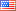# Revision history [back]

### list of random non-zero elements

I was wondering if there was a short way to generate lists of random non-zero elements of GF(q) ? What I have so far is:

 L=[];
for i in range(10):
a=GF(25).random_element();
while a==0:
a=GF(25).random_element();
L.append(a);2 NoneJohn Palmieri5583 ●18 ●50 ●125 http://www.math.washin...

### list of random non-zero elements

I was wondering if there was a short way to generate lists of random non-zero elements of GF(q) ? What I have so far is:

  L=[];
for i in range(10):
a=GF(25).random_element();
while a==0:
a=GF(25).random_element();
L.append(a);